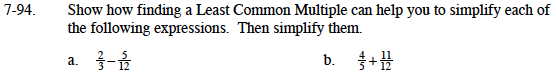### Home > MC1 > Chapter 7 > Lesson 7.2.5 > Problem7-94

7-94.What is the least common multiple of 3 and 12? How can this help you with this calculation?

Convert two-thirds to a fraction with a denominator of 12.

$\left ( \frac{2}{3} \right )\left ( \frac{4}{4} \right )=\frac{8}{12}$

$\frac{8}{12}-\frac{5}{12}=\frac{3}{12}=\frac{1}{4}$
$\frac{1}{4}$